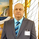612 obejrzeń
This script utilizes this concept. Instead of weighting by volume , it weights by amount of price action on every close price of the rolling window. I assume it can be used as an additional reference point for price mode and price antimode.

it is directly connected with Market (not volume ) profile, or TPO charts.

The algorithm:
1) takes a rolling window of, for example, 50 data points of close prices:
2) for each of this closing prices, the algorithm will check how many bars touched this close price.
3) then: sum of datapoints * weights/sum of weights

Since the logic is implemented in pretty non-efficient way, the script sometimes can take time to make calculations. Moreover, it calculates the centroid taking into account only close prices, not every tick. of a given rolling window That's why it's still experimental.

## KomentarzeI am assuming that in the pine_centroid function, x and y will be the close and the time?
Thanks.
Odpowiedzsyracusepro
@syracusepro, yes, take a look at the line 32 where the function is finally called. The third argument is "src", and the fourth is "length" .
Odpowiedzsyracusepro
@syracusepro, Ok, imagine you chose 100 close prices in script's settings. Now there is a function, I called it "density", on line 11. Now this function will take each of these 100 close prices and check how many bars touched each of these close prices within the rolling window. Hope it does make sense, if not, imma draw a picture n drop it here.
Odpowiedz